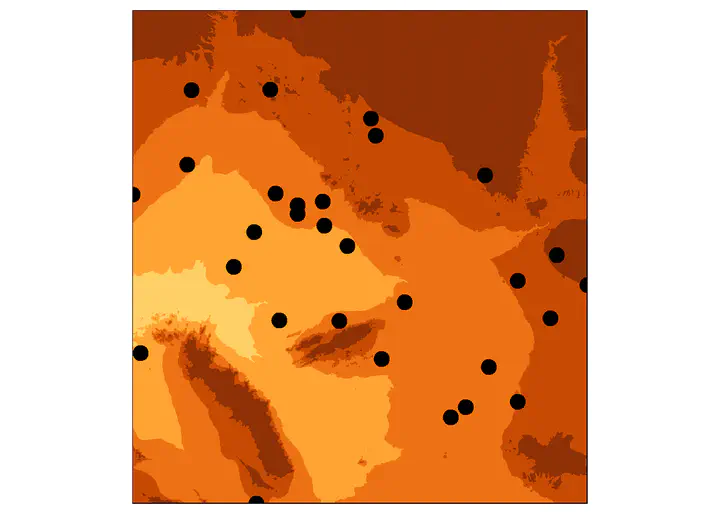# Function to Extract ElevationSimilar to the Planet workflow. In this test case, I downloaded 1/3 arc second DEMs from the USGS NED (in Maricopa), keep them in a folder, wrote a function in R to mosaic whatever is in that folder, then join to properties.

Prelims

``````#call packages
library(sf)
library(raster)
library(rgdal)
library(dplyr)
library(tmap)
library(tmaptools)
``````

# Example

Read in feature you want to join raster values to

``````MaricopaSample<- read_sf("MaricopaSample.shp")
``````

As an example, consider a sample of Maricopa County properties (as points) and a single USGS 1/3 arc second DEM raster. We can clearly see that DEM tiles are smaller than the study area.

``````PointMap1<-#make map

tm_shape(stack("USGS_13_n34w112_20210301.tif"), bbox= bb(MaricopaSample))+
tm_raster(breaks = c(0, 200, 250, 300, 350, 400, 500, Inf))+

tm_shape(MaricopaSample)+
tm_dots( size=1 )+

tm_layout(legend.show = FALSE)

PointMap1
``````

There are actually 8 DEMs that intersect with Maricopa, two of which cover this sample. This makes joining elevation (or any raster information) to properties over a large area difficult.

``````PointMap2<-#make map

tm_shape(stack("USGS_13_n34w112_20210301.tif"), bbox= bb(MaricopaSample))+
tm_raster(breaks = c(0, 200, 250, 300, 350, 400, 500, Inf))+

tm_shape(stack("USGS_13_n34w113_20130911.tif"), bbox= bb(MaricopaSample))+
tm_raster(breaks = c(0, 200, 250, 300, 350, 400, 500, Inf))+

tm_shape(MaricopaSample)+
tm_dots(size=1)+

tm_layout(legend.show = FALSE)

PointMap2
``````

# Join raster with user defined function

This function performs a join with many rasters by first mosaicing them together. The output is in the same format as the feature you join to (e.g. point, polygon).

``````# 2 inputs: the location of rasters to be mosaiced, and the shapefile (unit of analysis) you want to join raster values to
RasterJoin<-function(RasterLocation,UOA){

# make list of file names that are rasters. Note to change the pattern argument if rasters are not .tif. Alternatively you can remove the pattern argument if the file location only stores relevant raster files to be mosaiced.
current.list <- list.files(path=paste(RasterLocation), full.names=TRUE, pattern = ".tif")

# read in raster files as a raster stack
raster.list<- lapply(current.list, stack)

# clear user defined names if present
names(raster.list) <- NULL

# this tells the mosaic function to average any overlapping pixels
raster.list\$fun <- mean

# mosaic list using the mosaic function the raster package
Mosaic <- do.call(mosaic, raster.list)

# ensure joining units have a coordinate system
UOA<-st_transform(UOA, crs = 3857)

# match coordinate system of the mosaiced raster
Mosaic<-projectRaster(Mosaic, crs = crs(UOA))

# extract (join) raster values to points or polygons.
ex <- extract(Mosaic,
UOA,
fun=mean, #If polygons, take the mean raster values (change to sum or other function if desired)
na.rm=TRUE,
df=TRUE)

ex <-cbind(UOA, ex)

return(ex)
}
``````

#### Run RasterJoin Function

``````MaricopaElevation<-RasterJoin(RasterLocation= getwd(), #relative working directory, or change "getwd()" to raster folder
UOA= MaricopaSample) # feature we want to join to
``````

#### Export Features

``````st_write(MaricopaElevation,#export as shapefile
paste(getwd(),"/","MaricopaElevation.shp",sep="")) #send to working directory (change to relevant folder and file name if different)

write.csv(MaricopaElevation, #export as csv
paste(getwd(),"/","MaricopaElevation.csv",sep="")) #send to working directory (change to relevant folder and file name if different)
``````

#### Map Example of the Joined Elevation

Note that the variable indicating elevation in the new data is “layer” which you can change to some appropriate heading

``````map3<-#make map
tm_shape(MaricopaElevation)+
tm_dots(col="layer", palette = "Reds", border.col = NULL, breaks = c(0, 200, 250, 300, 350, 400, 500, Inf), size = 1)

map3
``````